# MCQs on Electrical Machines

##### Page 39 of 114. Go to page 1 2 3 4 5 6 7 8 9 10 11 12 13 14 15 16 17 18 19 20 21 22 23 24 25 26 27 28 29 30 31 32 33 34 35 36 37 38 39 40 41 42 43 44 45 46 47 48 49 50 51 52 53 54 55 56 57 58 59 60 61 62 63 64 65 66 67 68 69 70 71 72 73 74 75 76 77 78 79 80 81 82 83 84 85 86 87 88 89 90 91 92 93 94 95 96 97 98 99 100 101 102 103 104 105 106 107 108 109 110 111 112 113 114
01․ Transformer action requires a
constant magnetic flux.
increasing magnetic flux.
alternating magnetic lux.
alternating electric flux.

A basic transformer contains two magnetically coupled coils on a common core. If an alternating voltage is given to one winding (known as primary), due to electromagnetic and action mutual induction, there is a voltage induced in the secondary winding where load can be connected. The energy transfer from one winding to another is entirely through magnetic medium and known as transformer action based on the principle of electromagnetic induction proposed by Faraday. Therefore, transformer action requires an alternating or time varying magnetic flux to transfer power from primary side to secondary side since induced emf in the winding is due to flux linkage.

02․ For an ideal transformer the winding should have
maximum resistance on primary side and least resistance on secondary side
minimum resistance on primary side and maximum resistance on secondary side
equal resistance on primary and secondary side
no ohmic resistance on either side

For an ideal transformer the losses should be zero on both sides. Therefore the ohmic resistance on either side of the transformer should be equal to zero.

03․ Which of the following statements is/are correct statements?
EMF per turn in HV winding is more than EMF per turn in LV winding
EMF per turn in HV winding is less than EMF per turn in LV winding
EMF per turn in both the windings are equal
all of the above

In a transformer primary volt-ampere is equal to secondary volt-ampere and primary ampere turns are also equal to secondary ampere turns. So, EMF per turn in both the windings is equal. Total induced emf on both sides depends on the number of turns, flux and frequency. If number of turns on secondary is more than primary, then emf induced in the secondary will be more than primary side and vice versa, but the emf per turn in both the windings is equal.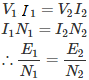04․ The core flux in transformer depends mainly on
supply voltage
supply voltage and frequency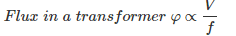Therefore core flux in transformer depends mainly on supply voltage and frequency.

05․ If the applied voltage of a certain transformer is increased by 50% and the frequency is reduced by 50%, the maximum flux density will (assuming that the magnetic circuit remains unsaturated)
changes to three times the original value
changes to 1.5 times the original value
changes to 0.5 times the original value
remain the same as the original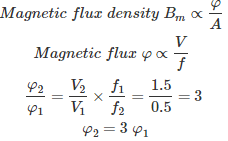.

06․ The low voltage winding of a 400/230 V single phase 50 Hz transformer is to be connected to keep the magnetization current at the same level in both the cases the voltage at 25 Hz should be
230 V
460 V
115 V
65 V

To maintain the magnetization current at the same level, flux φ should be same i.e V/f ratio should be same.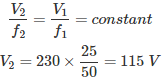07․ A single phase transformer has specifications as 250 KVA, 11000 V/415 V, 50 Hz. What are the values of primary and secondary currents?
primary = 22.7 A, secondary = 602.4 A
primary = 602 A, secondary = 22.7 A
primary = 301 A, secondary = 22.7 A
primary = 11.4 A, secondary = 301 A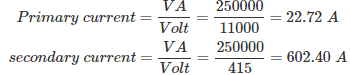08․ R1 is the resistance of the primary winding of the transformer. The turn ratio in terms of primary to secondary is K. Then the equivalent resistance of the primary referred to secondary is
R1 /K
K²R1
R1 /K²
K×R1

By changing of primary parameters to secondary or vice versa it does not change the performance of circuit. So that we are taking power rating are equal in before and after referring.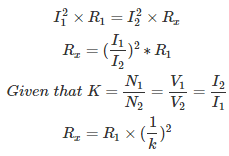Therefore, the equivalent resistance of the primary referred to secondary = R1 /K²

09․ A 5 KVA transformer has a turns ratio of N1/N2 = 10. The impedance of primary winding is 3+j5 ohms while that of secondary winding is 0.5+j0.8 ohms. The impedance of transformer when referred to primary will be
3.5+j5.8 ohms
8+j13 ohms
53+j85 ohms
3.05+j5.08 ohms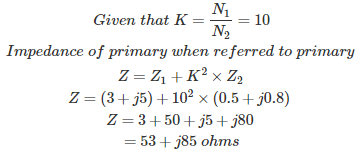10․ Voltage regulation of transformer is given by
(E2 - V2) / V2
(E2 - V2) / E2
(V2 - E2) / V2
(V2 - E2) / E2

Voltage regulation is defined as the change in terminal voltage when the rated load at a given power factor is disconnected across the terminals expressed in terms of rated secondary voltage.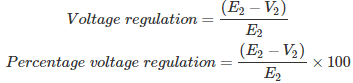<<<3738394041>>>